TitleCollege Algebra
Tutorial 22: Linear Inequalities

WTAMU > Virtual Math Lab > College AlgebraLearning Objectives

 After completing this tutorial, you should be able to: Use the addition, subtraction, multiplication, and division properties of  inequalities to solve linear inequalities. Solve linear inequalities involving absolute values. Write the answer to an inequality using interval notation. Draw a graph to give a visual answer to an inequality problem.Introduction

 In this tutorial we will be looking at solving linear inequalities.  When solving linear inequalities, we use a lot of the same concepts that we use when solving linear equations (shown in Tutorial 14: Linear Equations in One Variable).  Basically, we still want to get the variable on one side and everything else on the other side by using inverse operations.  The difference is, when a variable is set equal to one number, that number is the only solution.  But, when a variable is less than or greater than a number, there are an infinite number of values that would be a part of the answer.  We will also revisit the definition of absolute value and how it applies to inequalities.  If you need a review on absolute values go to Tutorial 21: Absolute Value Equations.   You never know when you will need to know about inequalities, so you better get started.Tutorial

 Inequality Signs

 Read left to right: a < b    a is less than b a < b   a is less than or equal to b a > b     a is greater than b a > b    a is greater than or equal to b

 Interval Notation

Interval notation is a way to notate the range of values that would make an inequality true.  There are two types of intervals, open and closed (described below), each with a specific way to notate it so we can tell the difference between the two.

Note that in the interval notations (found below), you will see the symbol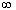, which means infinity

 Positive infinity (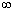) means it goes on and on indefinitely to the right of the number - there is no endpoint on the right.  Negative infinity (-) means it goes on and on indefinitely to the left of the number - there is no endpoint to the left.  Since we don’t know what the largest or smallest numbers are, we need to use infinity or negative infinity to indicate there is no endpoint in one direction or the other.

In general, when using interval notation, you always put the smaller value of the interval first (on the left side), put a comma between the two ends, then put the larger value of the interval (on the right side).  You will either use a curved end ( or ) or a boxed end [ or  ], depending on the type of interval (described below).

If you have either infinity or negative infinity on either end, you always use a curve for that end. This will indicate that there is no definite endpoint in that direction,  it keeps going and going.

 Open Interval

 An open interval does not include where your variable is equal to the endpoint. To indicate this, we use a curved end as shown below.

 Inequality Interval Notation for Open Intervals x > a (a,) x < a (-, a)

 When you graph an open ended end point, you use the same curved end (  or  ) on the graph as you do in the interval notation.   Also, darken in the part of the graph that is the solution.  For example,

 Inequality Interval Notation for  Open Intervals Graph x > 4 (4,)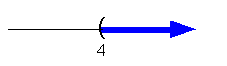x < 4 (-, 4)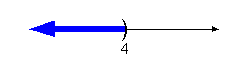Closed Interval

 A closed interval includes where your variable is equal to the endpoint. To indicate this, we use a boxed end as shown below.  As mentioned above, even though a is included and has a boxed end, if it goes to either infinity or negative infinity on the other end, we will notate it with a curved end for that end only!

 Inequality Interval Notation for Closed Intervals x > a [a,) x < a (-, a]

 When you graph a closed ended end point, you use the same boxed end [  or  ] on the graph as you do in the interval notation.   Also, darken in the part of the graph that is the solution.  For example,

 Inequality Interval Notation for  Closed Intervals Graph x > 4 [4,)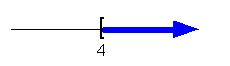x < 4 (-, 4]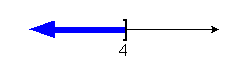Combining Open and Closed Intervals

 Sometimes one end of your interval is open and the other end is closed.  You still follow the basic ideas described above.  The closed end will have a [   or ] on it’s end and the open end will have a ( or ) on its end.

 Inequality Interval Notation for Combining Open and Closed Intervals a < x < b (a, b] a < x < b [a, b)

 Inequality Interval Notation for  Combining Open and  Closed Intervals Graph 3 < x < 6 [3, 6)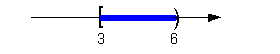Addition/Subtraction Property for Inequalities If a < b, then a + c < b + c If a < b, then a - c < b - c

 In other words, adding or subtracting the same expression to both sides of an inequality does not change the inequality.Example 1: Solve, write your answer in interval notation and   graph   the solution set: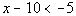.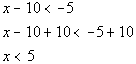Interval notation: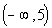Graph: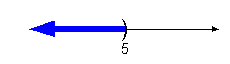*Inv. of sub. 10 is add. 10     *Open interval indicating all values less than 5 *Visual showing all numbers less than 5 on the number line

 Note that the inequality stayed the same throughout the problem.  Adding or subtracting the same value to both sides does not change the inequality. The answer 'x is less than 5' means that if we put any number less than 5 back in the original problem, it would be a solution (the left side would be less than the right side).  As mentioned above, this means that we have more than just one number for our solution, there are an infinite number of values that would satisfy this inequality. Interval notation: We have an open interval since we are not including where it is equal to 5.   x is less than  5, so 5 is the largest value of the interval, so it goes on the right.  Since there is no lower endpoint (it is ALL values less than 5), we put the negative infinity symbol on the left side.  The curved end on 5 indicates an open interval.  Negative infinity always has a curved end because there is not an endpoint on that side. Graph:  We use the same type of notation on the endpoint as we did in the interval notation,  a curved end.  Since we needed to indicate all values less than 5, the part of the number line that was to the left of 5 was darkened.Example 2: Solve, write your answer in interval notation and graph the solution set: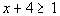.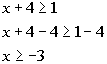Interval notation: [-3,) Graph: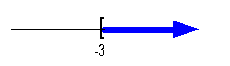*Inv. of add 4 is sub. 4   *Closed interval indicating all values greater than or = -3 *Visual showing all numbers greater than or = to -3 on the number line.

 Note that the inequality stayed the same throughout the problem.  Adding or subtracting the same value to both sides does not change the inequality. The answer 'x is greater than or equal to -3' means that if we put any number greater than or equal to -3 back in the original problem, it would be a solution (the left side would be greater than or equal to the right side).  As mentioned above, this means that we have more than just one number for our solution, there are an infinite number of values that would satisfy this inequality. Interval notation: We have a closed interval since we are including where it is equal to -3.   x is greater than or equal to -3, so -3 is our smallest value of the interval, so it goes on the left.  Since there is no upper endpoint (it is ALL values greater than or equal to -3), we put the infinity symbol on the right side.  The boxed end on -3 indicates a closed interval.  Infinity always has a curved end because there is not an endpoint on that side. Graph:  We use the same type of notation on the endpoint as we did in the interval notation,  a  boxed end.  Since we needed to indicate all values greater than or equal to -3, the part of the number line that was to the right of -3 was darkened.

 Multiplication/Division Properties for Inequalities when multiplying/dividing by a positive value If a < b  AND  c is positive, then   ac < bc If a < b  AND  c is positive, then   a/c < b/c

 In other words, multiplying or dividing the same POSITIVE number to both sides of an inequality does not change the inequality.Example 3: Solve, write your answer in interval notation and graph the solution set: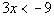.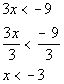Interval notation: (-, -3) Graph: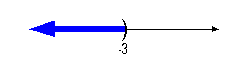*Inv. of mult. by 3 is div. by 3     *Open interval indicating all values less than -3 *Visual showing all numbers less than -3 on the number line

 Note that the inequality sign stayed the same direction.  Even though the right side was a -9, the number we were dividing both sides by, was a positive 3.  Multiplying or dividing both sides by the same positive value does not change the inequality. Interval notation: We have an open interval since there we are not including where it is equal to -3.   x is less than  -3, so -3 is our largest value of the interval, so it goes on the right.  Since there is no lower endpoint (it is ALL values less than -3), we put the negative infinity symbol on the left side.  The curved end on -3 indicates an open interval.  Negative infinity always has a curved end because there is not an endpoint on that side. Graph:  We use the same type of notation on the endpoint as we did in the interval notation,  a curved end.  Since we needed to indicate all values less than -3, the part of the number line that was to the left of -3 was darkened.

 Multiplication/Division Properties for Inequalities when multiplying/dividing by a negative value If a < b  AND  c is negative, then   ac > bc If a < b  AND  c is negative, then   a/c > b/c

 In other words, multiplying or dividing the same NEGATIVE number to both sides of an inequality reverses the sign of the inequality. The reason for this is, when you multiply or divide an expression by a negative number, it changes the sign of that expression.  On the number line, the positive values go in a reverse or opposite direction than the negative numbers go, so when we take the opposite of an expression,  we need to reverse our inequality to indicate this.Example 4:  Solve, write your answer in interval notation and graph the solution set: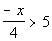.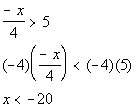Interval notation: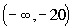Graph: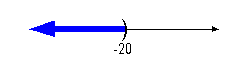*Inv. of div. by -4 is mult. by -4, so reverse inequality sign     *Open interval indicating all values less than -20 *Visual showing all numbers less than -20 on the number line

 I multiplied by a -4 to take care of both the negative and the division by 4 in one step.  In line 2, note that when I did show the step of multiplying both sides by a -4, I reversed my inequality sign. Interval notation: We have an open interval since we are not including where it is equal to -20.   x is less than  -20, so -20 is our largest value of the interval, so it goes on the right.  Since there is no lower endpoint (it is ALL values less than -20), we put the negative infinity symbol on the left side.  The curved end on -20 indicates an open interval.  Negative infinity always has a curved end because there is not an endpoint on that side. Graph:  We use the same type of notation on the endpoint as we did in the interval notation,  a curved end.  Since we needed to indicate all values less than -20, the part of the number line that was to the left of -20 was darkened.Example 5:  Solve, write your answer in interval notation and graph the solution set: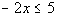.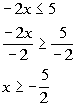Interval notation: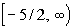Graph: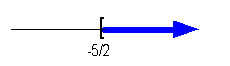*Inv. of mult. by -2 is div. by -2, so reverse inequality sign     *Closed interval indicating all values greater than or = -5/2 *Visual showing all numbers greater than or = -5/2 on the number line

 In line 2, note that when I did show the step of dividing both sides by a -2, that I reversed my inequality sign. Interval notation: We have a closed interval since we are including where it is equal to -5/2.   x is greater than or equal to -5/2, so -5/2 is our smallest value of the interval so it goes on the left.  Since there is no upper endpoint (it is ALL values greater than or equal to -5/2), we put the infinity symbol on the right side.  The boxed end on -5/2 indicates a closed interval.  Infinity always has a curved end because there is not an endpoint on that side. Graph:  We use the same type of notation on the endpoint as we did in the interval notation,  a  boxed end.  Since we needed to indicate all values greater than or equal to -5/2, the part of the number line that was to the right of -5/2 was darkened.

 Strategy for Solving a Linear Inequality

Step 1:  Simplify each side if needed.

 This would involve things like removing ( ), removing fractions, adding like terms, etc.

Step 2:  Use Add./Sub. Properties to move the variable term on one side and all other terms to the other side.

Step 3: Use Mult./Div. Properties to remove any values that are in front of the variable.

Note that it is the same basic concept we used when solving linear equations as shown in Tutorial 14: Linear Equations in One Variable.Example 6: Solve, write your answer in interval notation and graph the solution set: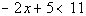.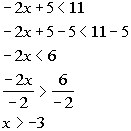Interval notation: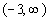Graph: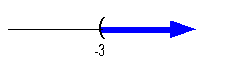*Inv. of add. 5 is sub. 5   *Inv. of mult. by -2 is div. both sides by -2, so reverse inequality sign   *Open interval indicating all values greater than -3   *Visual showing all numbers greater than -3 on the number line

 Interval notation: We have an open interval since we are not including where it is equal to -3.   x is greater than -3, so -3 is our smallest value of the interval so it goes on the left.  Since there is no upper endpoint (it is ALL values less than -3), we put the infinity symbol on the right side.  The curved end on -3 indicates an open interval.  Infinity always has a curved end because there is not an endpoint on that side. Graph:  We use the same type of notation on the endpoint as we did in the interval notation,  a curved end.  Since we needed to indicate all values greater than -3, the part of the number line that was to the right of -3 was darkened.Example 7:  Solve, write your answer in interval notation and graph the solution set: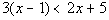.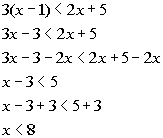Interval notation: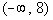Graph: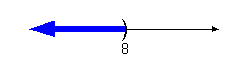*Distributive property *Get x terms on one side, constants on the other side *Inv. of sub. 3 is add. by 3       *Open interval indicating all values less than -1/2   *Visual showing all numbers less than -1/2 on the number line.

 Interval notation: Again, we have an open interval since we are not including where it is equal to 8.   This time  x is less than  8, so 8 is our largest value of the interval so it goes on the right.  Since there is no lower endpoint (it is ALL values less than 8), we put the negative infinity symbol on the left side.  The curved end on 8 indicates an open interval.  Negative infinity always has a curved end because there is not an endpoint on that side. Graph:  Again, we use the same type of notation on the endpoint as we did in the interval notation, a curved end.  Since we needed to indicate all values less than 8, the part of the number line that was to the left of 8 was darkened.Example 8:  Solve, write your answer in interval notation and graph the solution set: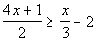.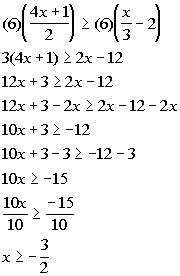Interval notation: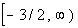Graph: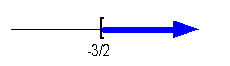*Mult. both sides by LCD of 6   *Get x terms on one side, constants on the other side   *Inv. of add. 3 is sub. by 3 *Inv. of mult. by 10 is div. by 10        *Closed interval indicating all values greater than or equal to -3/2   *Visual showing all numbers greater than or equal to -3/2 on the number line.

 Interval notation: This time we have a closed interval since we are including where it is equal to -3/2.   x is greater than or equal to -3/2, so -3/2 is our smallest value of the interval so it goes on the left.  Since there is no upper endpoint (it is ALL values greater than or equal to -3/2), we put the infinity symbol on the right side.  The boxed end on -3/2 indicates a closed interval.  Infinity always has a curved end because there is not an endpoint on that side. Graph:  Again, we use the same type of notation on the endpoint as we did in the interval notation,  a boxed end this time.  Since we needed to indicate all values greater than or equal to -3/2, the part of the number line that was to the right of -3/2 was darkened.

 Solving a Compound Inequality

 A compound linear inequality is one that has two inequalities in one problem.  For example, 5 < x + 3 < 10 or -1 < 3x < 5.  You solve them exactly the same way you solve the linear inequalities shown above, except you do the steps to three "sides" (or parts) instead of only two.Example 9:  Solve, write your answer in interval notation and graph the solution set: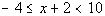.

 This is an example of an compound inequality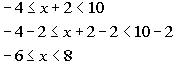Interval notation: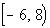Graph: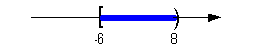*Inv. of add. 2 is sub. by 2 *Apply steps to all three parts      *All values between -6 and 8, with a closed interval at -6 (including -6)   *Visual showing all numbers between -6 and 8, including -6 on the number line.

 Interval notation: This time we have a mixed interval since we are including where it is equal to -6, but not equal to 8.   x is between -6 and 8, including -6, so -6 is our smallest value of the interval so it goes on the left and 8 goes on the right.   The boxed end on -6 indicates a closed interval on that side. A curved end on 8 indicates an open interval on that side.  Graph:  Again, we use the same type of notation on the endpoints as we did in the interval notation,  a boxed end on the left and a curved end on the right.  Since we needed to indicate all values between -6 and 8, including -6, the part of the number line that is in between -6 and 8 was darkened.

 Solving an Absolute Value Inequality

 Step 1:  Isolate the absolute value expression.

 If there is a constant that is on the same side of the inequality that the absolute value expression is but is not inside the absolute value, use inverse operations to isolate the absolute value.

 Step 2:  Use the definition of absolute value to set up the inequality without absolute values.

 A quick reminder, the absolute value measures the DISTANCE a number is away from the origin (zero) on the number line.  No matter if the number is to the left (negative) or right (positive) of zero on the number line, the DISTANCE it is away from zero is going to be positive. Hence, the absolute value is always positive, (or zero if you are taking the absolute value of 0).  If you need a review on absolute values, feel free to go to Tutorial 21: Absolute Value Equations.

 If d is POSITIVE and |x| < d, then -d < x < d  The graph below illustrates all the values on the number line whose distance would be less than d units away from 0.  It shows us why we set up the inequality, shown above, the way we do. If d is NEGATIVE and |x| < d, then there is no solution The absolute value is always positive, and any positive number is greater than any negative number, therefore it would be no solution.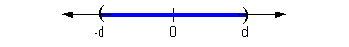If d is POSITIVE and |x| > d, then x < -d   OR   x > d The graph, shown below, illustrates all the values on the number line whose distance would be greater than d units away from 0.  It shows us why we set up the inequality, shown above, the way we do. If d is NEGATIVE and |x| > d, then x is all real numbers The absolute value is always positive, and any positive number is greater than any negative number, therefore all real numbers would work.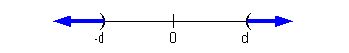Step 3:  Solve the linear inequalities set up in step 2.

 You will solve these linear inequalities just like the ones shown above.Example 10:  Solve, write your answer in interval notation and graph the solution set: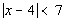.

 The absolute value expression is already isolated.

 Step 2:  Use the definition of absolute value to set up the inequality without absolute values. AND Step 3:  Solve the linear inequalities set up in step 2.

 The distance that the expression x - 4 is away from the origin needs to be less than 7.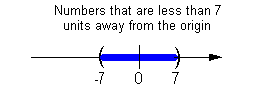All numbers between -7 and 7 are less than 7 units away from the origin.  So, the expression x - 4 needs to be between -7 and 7.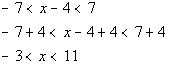Interval notation: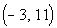Graph: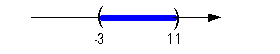*Inv. of sub. 4 is add. by 4 *Apply steps to all three parts   *All values between -3 and 11   *Visual showing all numbers between -3 and 11

 Interval notation: This time we have an open interval since we are not including either endpoint.  x is between -3 and 11, so -3 is our smallest value of the interval so it goes on the left and 11 goes on the right.   The curved end on both numbers indicate an open interval on both sides.  Graph:  Again, we use the same type of notation on the endpoints as we did in the interval notation,  a curved end on both ends.  Since we needed to indicate all values between -3 and 11,  the part of the number line that is in between -3 and 11 was darkened.Example 11:  Solve, write your answer in interval notation and graph the solution set: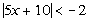.

 The absolute value expression is already isolated.

 Step 2:  Use the definition of absolute value to set up the inequality without absolute values. AND Step 3:  Solve the linear inequalities set up in step 2.

 Be careful, since the absolute value (the left side) is always positive, and positive values are always greater than negative values, the answer is no solution.  There is no value that we can put in for x that would make this inequality true.Example 12:  Solve, write your answer in interval notation and graph the solution set: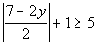.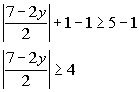*Inv. of add. 1 is sub. 1 *Abs. value exp. isolated

 Step 2:  Use the definition of absolute value to set up the inequality without absolute values. AND Step 3:  Solve the linear inequalities set up in step 2.

 The distance that the expression (7 - 2y)/2 is away from the origin needs to be greater than or equal to 4.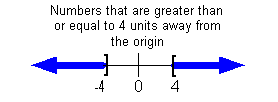All numbers that are less than or equal to - 4 OR greater than or equal to 4 are greater than or equal to 4 units away from the origin.  So the expression (7 - 2y)/2 needs to be less than or equal to  - 4 OR greater than or equal to 4.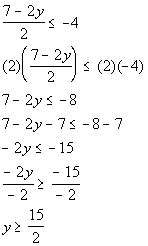OR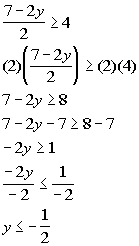Interval notation: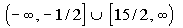Graph: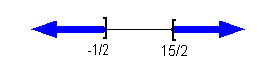*First inequality, where it is less than or = to -4 *Inv. of div. by 2 is mult. by 2       *Inv. of mult. by -2 is div. by -2, so reverse inequality sign             *Second inequality, where it is greater than or = to 4 *Inv. of div. by 2 is mult. by 2         *Inv. of mult. by -2 is div. by -2, so reverse inequality signs          *All values less than or = to -1/2 or greater than or = to 15/2    *Visual showing all numbers less than or = to -1/2 or greater than or = to 15/2

 Interval notation: This time we have two closed intervals since we are including the endpoints -1/2 and 15/2.  In the first interval, y is less than or equal to -1/2, so -1/2 is our largest value of the interval so it goes on the right.  Since there is no lower endpoint of that first interval, we put negative infinity on the left side.  The boxed end on -1/2 indicates a closed interval.  Infinity always has a curved end because there is not an endpoint on that side.  In the second, interval,  y is greater than or equal to 15/2, so 15/2 is our smallest value of the interval so it goes on the left.  Since there is no upper endpoint of that second interval, we put the infinity symbol on the right side.  The boxed end on 15/2 indicates a closed interval.  Infinity always has a curved end because there is not an endpoint on that side. Graph:  Again, we use the same type of notation on the endpoints as we did in the interval notation,  a boxed end on both y = -1/2 and y = 15/2.  Since we needed to indicate all values less than or equal to -1/2 OR greater than or equal to 15/2, the parts of the number line that are to the left of -1/2 and to the right of 15/2 were darkened.Example 13:  Solve, write your answer in interval notation and graph the solution set: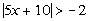.

 The absolute value expression is already isolated.

 Step 2:  Use the definition of absolute value to set up the inequality without absolute values. AND Step 3:  Solve the linear inequalities set up in step 2.

 Again, be careful - since the absolute value (the left side) is always positive, and positive values are always greater than negative values, the answer is all real numbers.  No matter what value you plug in for x, when you take the absolute value the left side will be positive.  All positive numbers are greater than -2.Practice Problems

 These are practice problems to help bring you to the next level.  It will allow you to check and see if you have an understanding of these types of problems. Math works just like anything else, if you want to get good at it, then you need to practice it.  Even the best athletes and musicians had help along the way and lots of practice, practice, practice, to get good at their sport or instrument.  In fact there is no such thing as too much practice. To get the most out of these, you should work the problem out on your own and then check your answer by clicking on the link for the answer/discussion for that  problem.  At the link you will find the answer as well as any steps that went into finding that answer.Practice Problems 1a - 1c: Solve, write your answer in interval notation and graph the solution set.

 1a.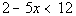(answer/discussion to 1a) 1b.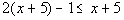(answer/discussion to 1b)

 1c.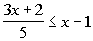(answer/discussion to 1c)Practice Problems 2a - 2d: Solve, write your answer in interval notation and graph the solution set.

 2a.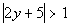(answer/discussion to 2a) 2b.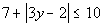(answer/discussion to 2b)

 2c.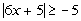(answer/discussion to 2c) 2d.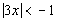(answer/discussion to 2d)Need Extra Help on these Topics?

The following are webpages that can assist you in the topics that were covered on this page:

 http://www.wtamu.edu/academic/anns/mps/math/mathlab/int_algebra/int_alg_tut10_linineq.htm This website helps you with linear inequalities. http://www.sosmath.com/algebra/inequalities/ineq01/ineq01.html This website helps you with linear inequalities. http://www.math.com/school/subject2/lessons/S2U3L4DP.html This website helps you with linear inequalities.

Go to Get Help Outside the Classroom found in Tutorial 1: How to Succeed in a Math Class for some more suggestions.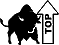Videos at this site were created and produced by Kim Seward and Virginia Williams Trice.
Last revised on Dec. 17, 2009 by Kim Seward.